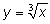Latoyia R.

The graph of is shifted 1 unit down and 4 units left. Which equation represents the new graph?

The graph ofis shifted 1 unit down and 4 units left. Which equation represents the new graph?